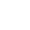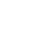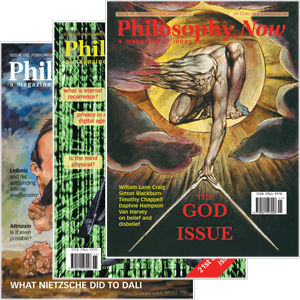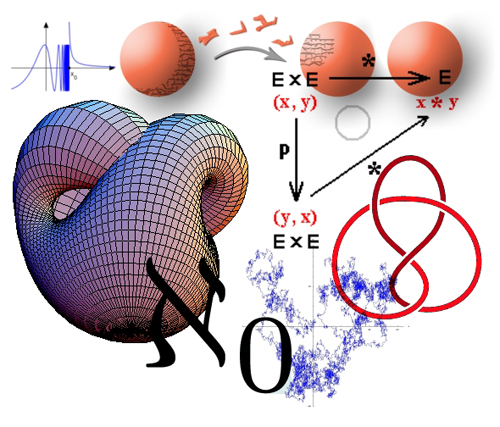# Solving the Mystery of Mathematics

### Jared Warren says, think of a number. What exactly are you thinking of?

Mathematics is mysterious. By this I don’t just mean that there are many difficult, unsolved mathematical questions. I mean instead that mathematics itself, as a subject, and as an activity, is mysterious. Our familiarity with elementary mathematics sometimes lets us lose sight of this. This is probably a good thing most of the time. Reflecting on the nature of mathematics is no aid to mathematical problem-solving. But doing mathematics is one thing, understanding the nature of mathematics is another. The first task is mathematical, the second philosophical.

Why is mathematics mysterious? Because it is difficult to see how mathematics fits into our picture of the world. Mathematics concern specific truths and falsehoods: it is true that 1+1=2 and it is false that 1+1=3. It is also true that there is an empty set (a set of things with nothing in it), and false that some sets are members of themselves. These are truths and falsehoods in arithmetic and set theory respectively, which are branches of pure mathematics. Pure mathematics contrasts with applied mathematics, as in applied to the physical world. There are questions about how mathematics manages to apply to the world in all of the unexpected ways it does. The physicist Eugene Wigner famously said that mathematics was ‘unreasonably’ effective at applying to the world. However, the puzzles I am raising here are even more basic; they concern the most fundamental aspects of pure mathematics itself.

The truths of pure mathematics are quite unlike ordinary truths. For instance, pure mathematical truths are eternal : they have always been and will always be true. It was true at the Big Bang that 1+1=2, and it will still be true in a trillion years. They are also necessary – they would remain true no matter the state of the world. In a world with no humans, it would still be true that 1+1=2. And they are objective – they do not depend on anyone’s whims, wishes, or will. If we encounter Martians, and they say that 1+1=3, we will know that we are failing to communicate with them, or that Martians are liars.

Crucially, mathematics also concerns distinctively mathematical objects : numbers, points, sets, functions, groups, and more. These objects are non-physical. They are not a part of physical reality the way that bodies, tables, and planets are. Nobody has ever tripped over a number, nor seen one in a telescope. Mathematical objects are not out there in any sense of the term. Yet they also don’t seem to be in here, in the way that mental images, thoughts, and emotions are. Yet somehow, someway, we know many mathematical truths, with certainty. And we expect to discover many more.

Clearly, our mathematical knowledge comes to us in special ways. No experiments are performed in the pure mathematics classroom. No data catalogued, no observations made. Most of our usual methods of finding things out are absent. Instead, mathematical knowledge comes by way of rigorous logical proofs from basic principles – the axioms, like ‘one is a number’ or ‘all right angles are the same’– which are taken to be self-evidently true.

These features make mathematics mysterious. Our challenge is to fit mathematics into a reasonable account of the world. Only then will we truly understand mathematics philosophically. This challenge has obsessed some of history’s greatest mathematicians and philosophers, but no clear answer has yet emerged.Mathematics general Petr Glivický 2008 public domain

## Metaphysical Miscalculations

There was once a promising approach to understanding the metaphysics of mathematics: conventionalism. This is the idea that mathematical truths are a byproduct of our linguistic conventions: they simply depend upon how we use language. Today conventionalism is almost universally rejected by philosophers of mathematics. Yet despite current fashions, I think conventionalism can be resuscitated. To set the stage for this, let’s see why the most obvious philosophical theories of mathematics badly fail.

Perhaps the most obvious philosophical approach to mathematics proceeds from an analogy. When you tell me that the cat is fat, you tell me something about the physical world. You tell me that a particular physical object, the cat, has a certain property, that of being fat. Perhaps mathematics likewise aims to describe an independently existing reality? Not physical reality, of course, but mathematical reality.

If this is right, mathematical claims are descriptive. When they accurately describe mathematical reality, (such as in 1+1=2) they are true. When they do not, they are false. So saying that three is a prime number is like saying that the cat is fat. In mathematical reality, the number three is an independently existing object, and being a prime number is a mathematical property.

According to this theory, the goal of mathematics is to correctly describe mathematical reality, and mathematics is like the ‘physics’ of this reality. But mathematical truth, as we’ve seen, is eternal, necessary, and objective. This view is usually called mathematical Platonism. Plato himself probably endorsed a version of it. According to Platonism, reality involves more than all facts about the physical world, it also includes independently-existing abstract eternal truths. Mathematical truths are part of the story. Indeed, here, what is called ‘mathematical reality’ is sometimes derisively called ‘Platonic heaven’.

The idea of independently-existing mathematical truths sits ill with our currently dominant understanding of the world, scientific naturalism. And truly, adding a special kind of fact to our picture of reality, whether mathematical, logical, moral, or theological, is not something we should do lightly. Perhaps if this were the only way to make sense of mathematics, it might be worth the metaphysical excess. But Platonism sits ill with the fact that we have mathematical knowledge.

To see this, suppose that Platonism is right, and also that ‘three is a prime number’ is true. It is true because it correctly describes mathematical reality. But how do we know it does? A connection to a supposed separate mathematical reality plays no role at all in generating this knowledge. If ‘independent mathematical reality’ vanished, we would still think that three is a prime. Likewise, if we were to stop thinking that three is a prime, it wouldn’t be because of better access to this supposed mathematical reality. No super telescope can ever point at Platonic mathematical heaven.

Knowledge requires some kind of connection between the facts known and our methods of investigation. I know that the cat is fat by way of a perceptual connection: I see the fat cat. But a connection need not be direct. Indirect connections often suffice. We know about black holes, though we never see them directly. But if Platonism is true, there is not even an indirect connection between mathematical reality and our mathematical methods. To reinstate a connection would require further departures from scientific naturalism – by positing supernatural faculties of mathematical insight, or the like. This is far too high a price to pay for a philosophical theory of mathematics. If we want to stay within scientific naturalism, Platonism must go. Take heart though, for rejecting Platonism is not the same as rejecting mathematics. Other approaches are possible.

At the other end of the spectrum are views that alter our basic assumptions about mathematical truth. Some such views deny that mathematics concerns truths at all. Others allow truth, but deny that mathematics is eternal, necessary, and objective. They claim instead that it is temporal, contingent, and subjective. Mathematics is invented, not discovered. According to these mathematical creationist views, we create mathematical objects and truth.

To make this approach plausible, the creationists point to the messy historical realities of mathematical practices. Wherever we look, we see human handprints, not crystalline purity. Mathematics was created by us, not handed down by the gods. It appears to be eternal, necessary, and objective, but these appearances are misleading, says the creationist. However, while reasoning like this is quite common in some circles, it is based on a simple confusion.

Obviously, mathematical concepts, words, and practices were invented by human beings. All human practices came into being at some time in history. And mathematical concepts can only exist where creatures are capable of conceptual thought. History, cognitive science, and psychology might all have light to shed on the development and implementation of mathematical concepts. I admit all of this, as everyone must. Yet admitting that the concept of number was invented is not the same as admitting that numbers themselves were invented. The concept of a planet was invented too, but planets were not (at least not by us).

This is what philosophers call a ‘use-mention’ confusion. Boston is a city in the Northeastern United States; but ‘Boston’ is a name. Boston has over a million residents, but it does not start with a ‘B’ (how can a city start with a ‘B’?). The name ‘Boston’ has no residents, but does start with a ‘B’. Likewise, the concept of a planet is not a planet. It does not orbit the sun. It is in the mind, not in space.

You might think that nobody has ever been confused by this. Unfortunately, you’d be wrong. The prominent sociologist of science Bruno Latour once argued that it is an anachronism to suggest that Pharaoh Ramses II died of tuberculosis because tuberculosis wasn’t ‘created’ until the nineteenth century. Obviously, this is absurd. The concept of tuberculosis was created in the nineteenth century, not the disease itself. Intermingling use and mention leads to silly confusions.

Likewise here. Arguments equating the creation of mathematical concepts to the creation of mathematical truths are mistaken. Our languages and practices and concepts were created, but mathematical truths and objects were not. Properly understanding mathematics requires avoiding this confusion.

## Core Conventional Cogitations

So we should reject both Platonism and creationism in mathematics. Mathematics isn’t descriptive in the way ordinary discourse is, but nor is it subjective. A better approach is available. We should understand mathematical claims on the model of conventionalism. Let me illustrate this with a few non-mathematical examples before explaining how to apply the idea to mathematics.

Consider the phrase, “Bishops move diagonally in chess.” This is true, but like mathematics, it doesn’t neatly fit into how we describe ordinary physical objects. The ability to move diagonally is not some inherent physical property of chess bishops. Chess bishops are not like planets or electrons. Instead, something counts as a bishop in a game of chess if it can move only diagonally within the game. The source of the truth of ‘Bishops move diagonally in chess’ is in the conventional rules of chess. These rules together constitute the game of chess. Fail to follow them, and you fail to play chess.

Many other conceptual truths are similarly conventional. Take the phrase, ‘bachelors are unmarried’. This is true, but it isn’t stating a fact about the physical world that we discovered scientifically. The rules of language use are the source of the truth that bachelors are unmarried: no-one married counts as a bachelor. There are many similar conceptual truths They can be generated explicitly, by stipulation or definition, and also implicitly, by following rules of language use. I call all such truths ‘conventional’. Conventional truths are familiar, and not at all mysterious.

Mathematical conventionalism is the view that mathematics should be understood in this way. Our conventional rules for using mathematical terms like ‘number’, ‘zero’, ‘plus’, ‘set’, ‘function’, etc, determine our mathematical concepts. Conventional rules are also the source of mathematical truth. With ordinary terms like ‘cat’, ‘table’ and ‘black hole’, the rules, together with the external facts, are the source of truth. In pure mathematics, there is no additional contribution from the external world. The conventional rules stand completely on their own. Mathematics doesn’t need an external Platonic world to be true.

I now want to argue that all of the mystery-generating features of mathematics can be neatly accommodated by conventionalism, without either the metaphysical excess of Platonism or the subjectivist confusion of creationism.

## Accounting for Mathematical Truth

Mathematical truth is mysterious because it is eternal, necessary, and objective. Platonism accounted for these features with a theory that made mathematical truths unknowable. Creationism denied these features entirely. Conventionalism can do better, provided some confusions are avoided. Let’s take each feature in turn.

Mathematical truth is eternal: truths of pure mathematics always were and always shall be. We can explain this in terms of our mathematical language, which makes no provision for variations of tense. If it was the case that 1+1=2 , then it is now the case, and will tomorrow be the case. Whether in the distant past or the far future, mathematical truth is exactly the same, because our mathematical language is atemporal. This is the sense in which mathematical truth is ‘eternal’. So conventionalism explains this apparently profound fact about mathematics without building metaphysical idols. The rules for using mathematical language suffice.

Mathematical truth is necessary: the truths of pure mathematics cannot be otherwise. This can also be explained using the conventions of mathematical language. Just as mathematical language is atemporal, it is also asubjunctive: there is no contrast within mathematical language between indicative claims (x is the case) and subjunctive claims (x would be the case). This means that if something is mathematically the case, then it also would be the case in any alternative state of affairs. Talk about any situation you like, whether actual or counterfactual, and mathematical truth is exactly the same. This is the sense in which mathematical truth is ‘necessary’. Once again, conventionalism explains an apparently puzzling feature without any metaphysical excess.

Mathematical truth is also objective: every perspective leads to the same mathematical truths. Conventionalism also explains this feature. For conventionalists, mathematical rules, and these rules alone, explain what it is we mean by mathematical terms such as ‘zero’, ‘number’, ‘set’, and ‘triangle’. Using alternative rules could, in a sense, change the truths, but only by changing the subject into something which isn’t our mathematics. Anyone who means what we mean by ‘number’ and the like must agree with us, ultimately, about mathematical truth. This explains why mathematics is objective. Yet again, conventionalism demystifies a puzzling feature.

Conventionalists admit that mathematics is eternal, necessary, and objective, while also admitting a degree of pluralism. Someone might play a game very much like chess, but with slightly different rules. Similarly, Martians might have mathematical practices very much like our own but with slightly different rules. This does not undermine the objectivity of mathematics, because the kind of pluralism I’m talking about wouldn’t amount to actual disagreement. We see this within our own practices. In Euclidean geometry the ‘parallel postulate’ – any line has a unique parallel line passing through a point not on the original line – is true. In non-Euclidean geometry it is not. To argue about whether it is ‘really true’ is silly: it depends on which language you’re operating in. Of course, you could sensibly argue about which version is true of physical space – but then you’d no longer be arguing about pure mathematics.

This sort of pluralism reigns in pure mathematics. But this shouldn’t make us fall back into assuming that when distinct mathematical practices seem to disagree, they’re discussing an independent mathematical reality. For conventionalists, there is no source of pure mathematical truth outside of linguistic conventions.

Conventionalism can also make sense of the existence of mathematical objects. Numbers, sets, and functions are not physical things. They are not made of matter. In fact, they aren’t made of anything at all. This is basically what we mean when we call them abstract objects, in contrast to physical objects. For claims like ‘tables exist’ or ‘black holes exist’ to be true, the physical world must meet certain conditions. That these claims require something specific about the physical world to be true, is why these objects are physical. With abstract objects, matters are different. The truth of ‘numbers exist’ places no conditions at all on the physical world. The rules of arithmetic allow you to conclude that one is a number. Since one is a number, something is a number, and so numbers exist. But once again, the rules of mathematics place no requirements at all on the physical world. Our mathematical rules themselves explain mathematical truth, such as the existence of numbers. So in this sense, existence in mathematics is metaphysically cheap.

This is not to say that mathematical existence is a special kind of existence. Tables, black holes, and numbers all exist in the same way. They are different kinds of things, not things with different ways of existing. Making sense of mathematical existence doesn’t require metaphysical pluralism about existence itself.

Could we just as easily derive the existence of God? No.What if we start speaking a language where ‘God exists’ is built into the rules for using the word ‘God’, in the way that ‘one is a number’ is built into the rules of basic arithmetic? This in fact changes the meaning of ‘God’. This putative proof of the existence of God fails for the same reason that you can’t prove the existence of God by renaming your dog ‘God’.

## How Mathematical Truth Works

Platonism makes mathematical knowledge impossible. Creationism makes it possible, but only by rejecting evident features of mathematical truth. In contrast, conventionalism can perfectly explain our growing body of mathematical knowledge without any revisions of our common understanding of it. In fact, conventionalism seems to be the only way of understanding why our mathematical practices are rational.

Rigorous mathematical practice starts from self-evident axioms. These are free. Other mathematical claims are known only when they have been rigorously (logically) proved from the axioms. In other words, mathematical practice looks exactly like you would expect it to look if conventionalism were true: setting out the self-evident starting principles, then building logically from these principles. This does not at all look like an attempt to describe an independent realm of extra-physical fact. Nor does it look like anything subjective. We know mathematical truths in the same way, and with the same certainty, that we know that bishops move diagonally, or that bachelors are unmarried. Conventionalism, and conventionalism alone, accounts for the certainty of mathematical knowledge.

Wait. Isn’t there a gap here? The supposed gap is between a concept like ‘2+2=4’ being true according to our practices, and it being actually true. If there is a gap, then mathematical knowledge is not yet explained. Fortunately, there is no gap. According to the rules of chess, bishops move diagonally. Similarly, when we are using arithmetical rules, 2+2=4. So there is no gap here to fill. Our rules determine what we mean, and what we mean alone determines what is mathematically true. You can be wrong about all sorts of individual mathematical claims, but changing how you use mathematical language often changes what you mean by those sentences. Rather, the conventions of our mathematical language fully determine both what we mean by mathematical terms and what is mathematically true in our language. So our knowledge of mathematics comes by way of proofs using our basic mathematical conventions. This is exactly what we find in the practice of working mathematicians. It is exactly what terrified students find in upper division mathematics classes in college. Conventionalism alone, of all the philosophical theories of mathematics, makes sense of the actual features of mathematical reasoning and knowledge-gathering.

## Coming to the Conventionalism Conclusion

You might now be wondering why everyone isn’t already a conventionalist about mathematics. Answering this question requires some philosophical history.

Primitive versions of mathematical conventionalism were already endorsed by philosophers as different from each other as Gottfried Leibniz (1646-1716) and David Hume (1711-1776). But the idea came into its own in the early twentieth century, after the development of modern mathematical logic allowed for more precise formulations of the view. For instance, a version of conventionalism was favored by the logical positivists, an influential group of philosophers, scientists, and mathematicians originally centered in Vienna. The positivists argued that all necessary truths, indeed any truths that can be known through reason alone, result from convention. They effectively argued that something was knowable from the armchair just in case it was true by convention. Logical, mathematical, and conceptual truths all illustrate this general convergence. The positivists embedded this approach to mathematics within a general philosophical approach to meaning which relied on their infamous ‘verification principle’: that a statement is meaningful only if it can be scientifically verified or refuted. But mathematical claims, being true or false by convention, were either automatically verified, or automatically refuted.

Using this framework, the positivists swept away the whole of traditional metaphysics and most of philosophy. The result was an exciting, apparently science-friendly vision of philosophy. Something of the excitement of the times was well-captured in A.J. Ayer’s Language, Truth, and Logic, published in 1936.

Unfortunately, it was too good to be true. Some of the problems concerned their treatment of mathematics, but most concerned other aspects of the overall framework. For example, critics said logical positivism was self-refuting, as the verification principle cannot itself be scientifically verified. Eventually logical positivism became a dead theory. But abandoning mathematical conventionalism with logical positivism would be jumping out of a seaworthy vessel. We can still be mathematical conventionalists without being verificationists about meaning. Further, we can be mathematical conventionalists without arguing that all necessary truths must be given the same treatment. Once this is realized, much of the uneasiness that some philosophers and mathematicians feel in their bones at the mere mention of conventionalism should start to wane.

On the contrary, conventionalism promises to explain mathematics without making strange or implausible claims about reality. Maybe naturalism ought to be overturned, but if so, not by our theorizing about logic and mathematics. Unlike most other philosophical theories of mathematics, conventionalism is compatible with scientific naturalism, perhaps even with physicalism.

Of course, a full defense of mathematical conventionalism would need to deal with many issues I haven’t even mentioned here, ranging from philosophical objections to technical challenges. A prominent problem is the difficulty of making sense of mathematical truth in light of Gödel’s incompleteness theorems. But instead of conclusively establishing the truth of conventionalism as a philosophical basis for mathematics, my more modest goal here has been to argue that conventionalism is worth considering.

Mathematics is mysterious. To solve the mystery we must fit mathematical practices into a reasonable picture of the world. Platonist and creationist attempts fail. Conventionalism is a better option. So, I am a conventionalist. You should consider being one too.